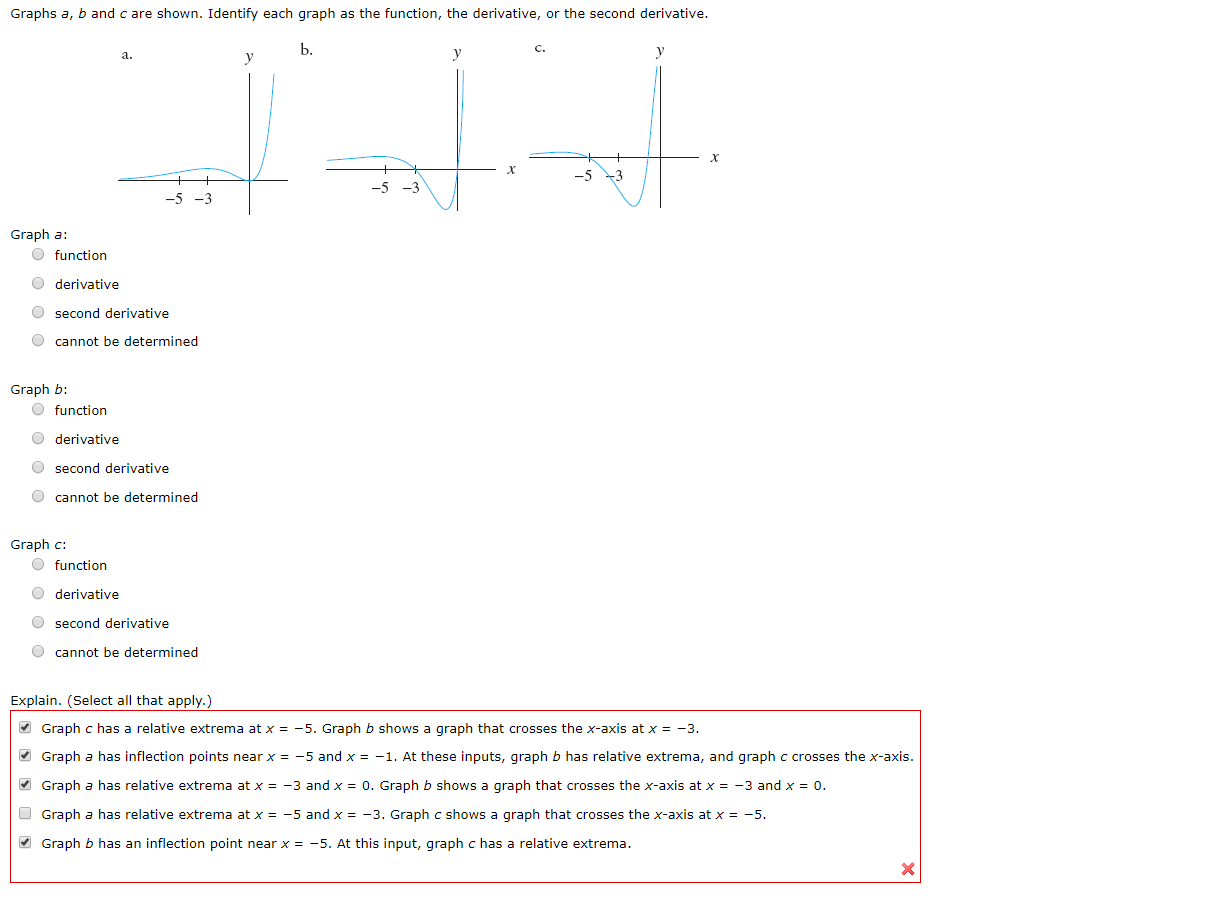# Graphs a, b and c are shown. Identify each graph as the function, the derivative, or the second derivative.Ь.уа.X-5 3-5-3Graph aO functionOderivativeOsecond derivativeOcannot be determinedGraph bO functionOderivativeO second derivativeOcannot be determinedGraph c:O functionOderivatiyeOsecond derivativeOcannot be determinedExplain. (Select all that apply.)Graph c has a relative extrema at x = -5. Graph b shows a graph that crosses the x-axis at x = -3.Graph a has inflection points near x-5 and x-1. At these inputs, qraph b has relative extrema, and graph c crosses the x-axis.-3 and x 0. Graph b shows a graph that crosses the x-axis at x = -3 and x = 0.Graph a has relative extrema at xGraph a has relative extrema at x-5 and x = -3. Graph c shows a graph that crosses the x-axis at x = -5.Graph b has an inflection point near x = -5. At this input, graph c has a relative extrema

Question
12 viewshelp_outlineImage TranscriptioncloseGraphs a, b and c are shown. Identify each graph as the function, the derivative, or the second derivative. Ь. у а. X -5 3 -5 -3 Graph a O function Oderivative Osecond derivative Ocannot be determined Graph b O function Oderivative O second derivative Ocannot be determined Graph c: O function Oderivatiye Osecond derivative Ocannot be determined Explain. (Select all that apply.) Graph c has a relative extrema at x = -5. Graph b shows a graph that crosses the x-axis at x = -3. Graph a has inflection points near x -5 and x -1. At these inputs, qraph b has relative extrema, and graph c crosses the x-axis. -3 and x 0. Graph b shows a graph that crosses the x-axis at x = -3 and x = 0. Graph a has relative extrema at x Graph a has relative extrema at x -5 and x = -3. Graph c shows a graph that crosses the x-axis at x = -5. Graph b has an inflection point near x = -5. At this input, graph c has a relative extrema fullscreen
check_circle

Step 1

Consider the give...

### Want to see the full answer?

See Solution

#### Want to see this answer and more?

Solutions are written by subject experts who are available 24/7. Questions are typically answered within 1 hour.*

See Solution
*Response times may vary by subject and question.
Tagged in

### Functions